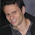## Friday, November 5, 2010

### An Encoding Trick

I use Vowpal Wabbit extensively at eHarmony, mainly for scalability purposes. While we are not Google or Yahoo scale, we do have a couple billion matches we've made recently that we like to model (at a major ad network, a billion impressions happen in a day or two; so we're about 2 to 3 orders of magnitude smaller in terms of data). Vowpal achieves high speed via the simplicity of gradient descent on a primal linear representation, but that means in practice one spends time trying nonlinear transformations of the raw data that improve model performance.

Here's a widely applicable encoding scheme that I've used for several different problems to improve performance. It is related to segmented regression (for statistical prediction folks) and also special order sets of type 2 (for operations research folks). We actually call it SOS2 encoding'' around the office.

In one dimension it works like this: one chooses a set of points for the variable, then for any input value two features are activated corresponding to the points bracketing the value, with weights corresponding to a linear interpolation between the two features. For example, for online dating physical distance between two people helps predict many quantities of interest. To encode the distance between two people for input to a model, I first take the $\log_2$ of the distance (ad-hoc intuited transform), then SOS2 encode using integral points. So, if two people have a distance difference of 72 miles, the two features output would be
LOG2_DISTANCE_6:0.83 LOG2_DISTANCE_7:0.19
Since this encoding is sparse it ends up interacting well with other variables (i.e., quadratic vowpal terms involving SOS2 encoded features can produce useful lift). In a two-dimensional problem I SOS2 encoded each dimension and then interacted them quadratically with some success. For higher dimensional problems I've gotten better mileage from other techniques (e.g., VQ).

1.Interesting technique! I think there's a typo: should the weight on LOG2_DISTANCE be .17 instead of .19?

Have you done much comparison with other methods of doing this type of thing? For example, what about just taking the closest point, and making the feature value the difference or percent change between the discretized value and the actual value?

1.Yes, that is a typo, thanks for noting (I'll preserve it so your comment continues to make sense ... exercise for the reader and all that ...)

Re: comparison, I haven't done anything systematic. So much of data science is heuristic because domain variability.

Note this technique corresponds to linear (quadratic with -q) spline interpolation. What you suggest sounds like piecewise linear (quadratic) but without enforcing continuity at the endpoints. If you suspect there should be jumps at your control points then maybe it would work better.